# Multi-particle collision dynamics

Multi-particle collision dynamics (MPC), also known as stochastic rotation dynamics (SRD), is a particle-based mesoscale simulation technique for complex fluids . Coupling of embedded particles to the coarse-grained solvent is achieved through molecular dynamics .

## Method of simulation

The solvent is modelled as a set of$N$ point particles of mass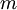$m$ with continuous coordinates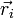$\vec{r}_{i}$ and velocities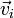$\vec{v}_{i}$. The simulation consists of streaming and collision steps.

During the streaming step, the coordinates of the particles are updated according to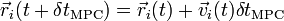$\vec{r}_{i}(t+\delta t_{\mathrm{MPC}}) = \vec{r}_{i}(t) + \vec{v}_{i}(t) \delta t_{\mathrm{MPC}}$

where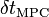$\delta t_{\mathrm{MPC}}$ is a chosen simulation time step which is typically much larger than a molecular dynamics time step.

After the streaming step, interactions between the solvent particles are modelled in the collision step. The particles are sorted into collision cells with a lateral size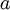$a$. Particle velocities within each cell are updated according to the collision rule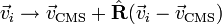$\vec{v}_{i} \rightarrow \vec{v}_{\mathrm{CMS}} + \hat{\mathbf{R}} ( \vec{v}_{i} - \vec{v}_{\mathrm{CMS}} )$

where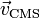$\vec{v}_{\mathrm{CMS}}$ is the centre of mass velocity of the particles in the collision cell and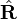$\hat{\mathbf{R}}$ is a rotation matrix. In two dimensions,$\hat{\mathbf{R}}$ performs a rotation by an angle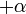$+\alpha$ or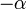$-\alpha$ with probability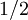$1/2$. In three dimensions, the rotation is performed by an angle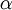$\alpha$ around a random rotation axis. The same rotation is applied for all particles within a given collision cell, but the direction (axis) of rotation is statistically independent both between all cells and for a given cell in time.

If the structure of the collision grid defined by the positions of the collision cells is fixed, Galilean invariance is violated. It is restored with the introduction of a random shift of the collision grid .